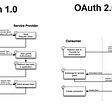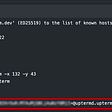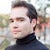# CoDEVIANT #22 — Linked Lists and Pyramid Schemes (I.P.)(10/12/20)

• Data
• Pointer to the next node
`let linkedList= { head: {   value: 1,   next: {      value: 2,      next: {         value: 3,         next: ...etc. etc.      }   } }}`
`class Node {  constructor(value) {    this.value = value;    this.next = null;  }}`
`let thing = new SinglyLinkedList()`
`class singlyLinkedList {  constructor() {    this.head = null    this.tail = null  }  ...}`
• We want to set the tail of the linked list
• We want to be able to set a new node before a particular node
• We want to be able to set a new node after a particular node
• We want to insert a node at a particular position in the linked-list
• We want to be able to remove a node with a particular value
• We want to know if a node in our linked list has a particular value
• We want to be able to remove a node in general
1. containsNodeWithValue(value)
`containsNodeWithValue(value) {  let node = this.head  while(node !== null && node.value !== value) {   node = node.next  }  return node !== null }`
`containsNodeWithValue(value) {...}`
`let node = this.head`
`containsNodeWithValue(value) {  let node = this.head  while(node !== null && node.value !== value) {    node = node.next  }}`
`return node !== null`
• We get to a node whose value equals the value that was passed in as the argument
• The node we get to is null (as in, we went beyond the length of the linked list itself because we couldn’t find a node with the value that was passed into the method)
`containsNodeWithValue(value) {  let node = this.head  while(node !== null && node.value !== value) {   node = node.next  }  return node !== null }`
`remove(node) {  if(node == this.head) {   this.head = this.head.next  }    //after settling issues of dealing with potential head/tail, then we remove the bindings (pointers) of the   //passed-in node argument  this.removeNodeBindings(node)  }`
`removeNode(node) { if(node === this.head) {   this.head = this.head.next }}`
`removeNodeBindings(node) {  let arrayOfNodes = []  let nodeToDelete = this.head  while(nodeToDelete !== null && nodeToDelete!== node) {   arrayOfNodes.push(nodeToDelete)   nodeToDelete = nodeToDelete.next  }  arrayOfNodes[arrayOfNodes.length - 1].next = node.next  node.next = null    }`
`removeNodeBindings(node) {  let arrayOfNodes = []}`
`removeNodeBindings(node) {  let arrayOfNodes = []  let nodeToDelete = this.head}`
`removeNodeBindings(node) {  //update pointer of node before this one  let arrayOfNodes = []let nodeToDelete = this.head  while(nodeToDelete !== null && nodeToDelete!== node) {   arrayOfNodes.push(nodeToDelete)   nodeToDelete = nodeToDelete.next  }}`
`arrayOfNodes[arrayOfNodes.length - 1].next = node.next`
`node.next = null`
`removeNodeBindings(node) {  let arrayOfNodes = []  let nodeToDelete = this.headwhile(nodeToDelete !== null && nodeToDelete!== node) {   arrayOfNodes.push(nodeToDelete)   nodeToDelete = nodeToDelete.next  }arrayOfNodes[arrayOfNodes.length - 1].next = node.nextnode.next = null    }`
`remove(node) {  if(node == this.head) {   this.head = this.head.next  }    this.removeNodeBindings(node)}`
`removeNodesWithValue(value) {  let node = this.head    while(node !== null) {   let nodeToRemove = node   node = node.next   if(nodeToRemove.value === value) {    this.remove(nodeToRemove)   } }}`
`removeNodesWithValue(value) {  let node = this.head}`
`removeNodesWithValue(value) {  let node = this.head  while(node !== null) {   let nodeToRemove = node   node = node.next  }}`
`removeNodesWithValue(value) {  let node = this.head  while(node !== null) {   let nodeToRemove = node   node = node.next   if(nodeToRemove.value === value) {     this.remove(nodeToRemove)   }  }}`
`insertBefore(node, nodeToInsert) {  if(nodeToInsert === this.head && nodeToInsert === this.tail)  {   return  }  this.remove(nodeToInsert)  nodeToInsert.next = node     let temp = this.head  let arrayOfNodes = []  while(temp !== null && temp !== node) {    arrayOfNodes.push(temp)    temp = temp.next  }  if(arrayOfNodes.length === 0) {    this.head = nodeToInsert  } else {    arrayOfNodes[arrayOfNodes.length - 1].next = nodeToInsert  }}`
`if(nodeToInsert === this.head && nodeToInsert === this.tail)  {   return }`
`this.remove(nodeToInsert)`
`let temp = this.headlet arrayOfNodes = []`
`while(temp !== null && temp !== node) {    arrayOfNodes.push(temp)    temp = temp.next}`
`if(arrayOfNodes.length === 0) {    this.head = nodeToInsert  } else {    arrayOfNodes[arrayOfNodes.length - 1].next = nodeToInsert  }`
`insertAfter(node, nodeToInsert) {   if(nodeToInsert === this.head && nodeToInsert === this.tail) {      return   }   this.remove(nodeToInsert)      nodeToInsert.next = node.next   if(node.next === null) {      this.tail = nodeToInsert   }   node.next = nodeToInsert}`
`if(nodeToInsert === this.head && nodeToInsert === this.tail) {      return   }`
`this.remove(nodeToInsert)`
`nodeToInsert.next = node.next`
`if(node.next === null) {      this.tail = nodeToInsert}`
`node.next = nodeToInsert`
`setHead(node) {  if(this.head === null) {    this.head = node    this.tail = node    return  }  this.insertBefore(this.head, node)}`
`if(this.head === null) {    this.head = node    this.tail = node    return  }`
`this.insertBefore(this.head, node)`
`setTail(node){  if(this.tail === null) {     this.setHead(node)     return  }  this.insertAfter(this.tail, node)}`
`if(this.tail === null) {     this.setHead(node)     return  }`
`if(this.tail === null) {     this.setHead(node)     return  }`
`insertAtPosition(position, nodeToInsert) {  if(position == 1) {    this.setHead(nodeToInsert)    return  }  let node = this.head  let currentPosition = 1  while(node != null && currentPosition != position) {    node = node.next    currentPosition++  }  if(node != null) {    this.insertBefore(node, nodeToInsert)  } else {    this.setTail(nodeToInsert)  }}`
`if(position == 1) {    this.setHead(nodeToInsert)    return  }`
`let node = this.headlet currentPosition = 1`
• node does not equal null
• currentPosition does not equal the position argument that was passed in
`while(node != null && currentPosition != position) {  node = node.next  currentPosition++}`
`if(node != null) {  this.insertBefore(node, nodeToInsert)} else {  this.setTail(nodeToInsert)}`

--

--

--

is a web developer, opera singer, actor, and lover of cats. (adrian-rosales.tech)

Love podcasts or audiobooks? Learn on the go with our new app.

## OAuth2.0 integration for webview## Applying filters and advanced filters on Data in Microsoft excel using VBA — Excel VBA## Why Dedicated Server is Best for Magento E-store Website## Debugging Github Actions## Create a GitHub Actions CI pipeline for a Python Package## Use MQTT with Raspberry Pi## 3 Reasons Why You Should Choose Bluehost To Host Websites.## Why an array index starts from 0 ?is a web developer, opera singer, actor, and lover of cats. (adrian-rosales.tech)

## Information About Placement Related Data For CSPIT IT Student## Relation with Elements## Happiness Project## Experience with My Group Project !